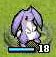# 星之一角

What have you found for these years?

## 2011-08-23

Last time I wrote this for creating permutations:

```permutation :: Eq a => [a] -> [[a]]
permutation [] = [[]]
permutation xs = do
x  <- xs
ys <- permutation (delete x xs)
return (x:ys)```
But after comparing to the one built in Ruby:
`array.permutation.to_a`
I found that the Haskell one is actually wrong!
The problem is, if `xs` has duplicated elements,
then we might pick one element but delete the other.
Consider input `"aba"`, and `x` would be iterating through
`'a'`, `'b'`, `'a'`, but what we delete would be `'a'`, `'b'`, and
first `'a'` again. Here's an updated version, more
complicated though :( The point is, we need to
know which exactly `x` and the remaining is.
So introducing a new function might be a must?
I am not sure though.
```split :: [a] -> [a] -> [(a, [a])]
split [] _ = []
split (x:xs) ys = (x, reverse ys++xs) : split xs (x:ys)

permutation :: Eq a => [a] -> [[a]]
permutation [] = [[]]
permutation xs = do
(y, ys) <- split xs []
zs      <- permutation ys
return (y:zs)```

#### 0 retries: